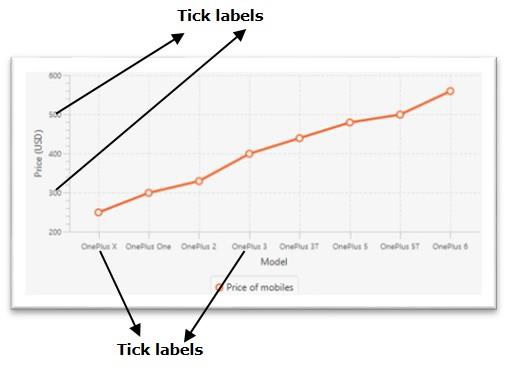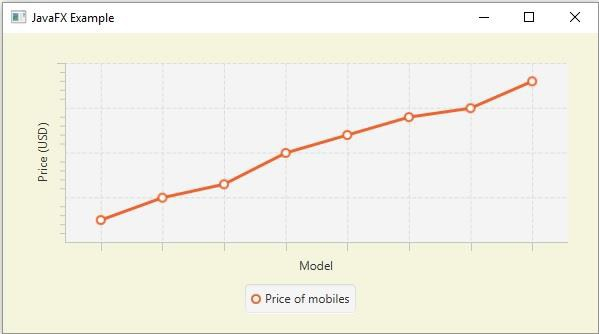# How to remove the tick labels in JavaFX XY charts?

The javafx.scene.XYChart class is the base class of all the charts that are plotted in an x-y pane. By instantiating the subclasses of this class you can create various XY charts namely − line chart, area chart, bar chart, pie chart, bubble chart, scatter chart, etc.

In the XY chart, the given data points are plotted on an XY plane. Along the x and y axes, you will have the tick marks and labels. The labels specify the names (or numbers) of the values.## Changing the color of the tick marks

The javafx.scene.chart.Axis class (abstract) is the base class of all the axes in XY charts. To create X and Y axes you need to instantiate subclasses of these classes

The NumberAxis class is used to create an axis for numerical values and the CategoryAxis class is used to create axis for string categories.

This class has a property named tickLabelVisible (boolean), it specifies whether to show the tick marks or not. You can set the value of this property using the setTickLabelVisible() method.

To remove the tick labels of an XY chart invoke this method by passing the boolean value false.

## Example

import javafx.application.Application;
import javafx.geometry.Insets;
import javafx.scene.Scene;
import javafx.stage.Stage;
import javafx.scene.chart.CategoryAxis;
import javafx.scene.chart.LineChart;
import javafx.scene.chart.NumberAxis;
import javafx.scene.chart.XYChart;
import javafx.scene.layout.StackPane;
public class RemovingTickLabels extends Application {
public void start(Stage stage) {
//Defining the x an y axes
CategoryAxis xAxis = new CategoryAxis();
NumberAxis yAxis = new NumberAxis(200, 600, 100);
//Setting labels for the axes
xAxis.setLabel("Model");
yAxis.setLabel("Price (USD)");
//Creating a line chart
LineChart linechart = new LineChart(xAxis, yAxis);
//Preparing the data points for the line
XYChart.Series series = new XYChart.Series();
//Setting the name to the line (series)
series.setName("Price of mobiles");
//Setting the data to Line chart
//Removing the tick labels
xAxis.setTickLabelsVisible(false);
yAxis.setTickLabelsVisible(false);
//Creating a stack pane to hold the chart
StackPane pane = new StackPane(linechart);
pane.setStyle("-fx-background-color: BEIGE");
//Setting the Scene
Scene scene = new Scene(pane, 595, 300);
stage.setTitle("JavaFX Example");
stage.setScene(scene);
stage.show();
}
public static void main(String args[]){
launch(args);
}
}

## Output